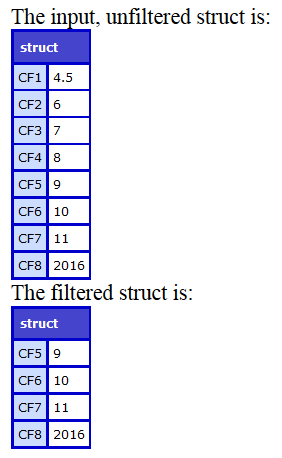#### Description

Filters the key-value pairs in a struct.

Filtered struct.

#### Syntax

`structFilter(struct,filter);`

#### History

ColdFusion 10: Added this function.

#### Parameters

Parameter

Description

struct

Name of the struct object.

filter

Inline function executed for each element in the array. Returns true if the key value pair in the struct has to be included in the resultant struct.

#### Example

```<cfscript>
myStruct={CF1=4.5,CF2=6,CF3=7,CF4=8,CF5=9,CF6=10,CF7=11,CF8=2016};
WriteOutput("The input, unfiltered struct is:");
WriteDump(myStruct);
myFilteredStruct=StructFilter(myStruct,function(key,value){
return value>8; //Return only those values >8
}
);
WriteOutput("The filtered struct is:");
WriteDump(myFilteredStruct);//Display the filtered struct
</cfscript>```

#### Output#### Using member function

```<cfscript>
myStruct={CF1=4.5,CF2=6,CF3=7,CF4=8,CF5=9,CF6=10,CF7=11,CF8=2016};
CFVersions=myStruct.filter(function(key,value){
return value>8;
});
WriteDump(CFVersions);
</cfscript>```Twitter™ and Facebook posts are not covered under the terms of Creative Commons.